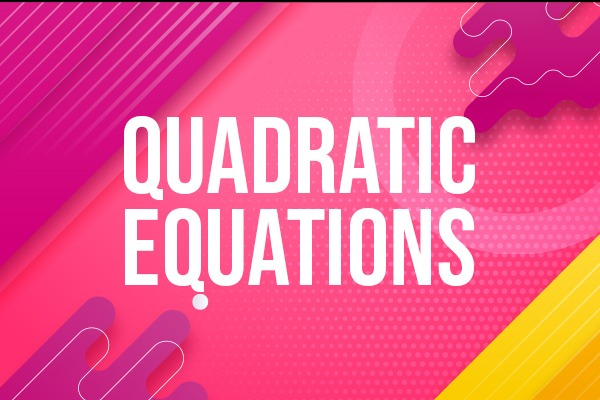Language: English

Instructors: PAVAN RC

₹1999 50.03% OFF

Why this course?

# Course Curriculum

 Presentations presentation by Smrithi and team (2:00) smrithi presentation 2 (6:00) spoorthi team presentation (9:00) smrithi presentation (9:00) vidith presentation (4:00) quadratic eq intro (8:00) Intro based quiz quadratic intro 2 (5:00) Intro 2 quiz Revision (3:00) Revision quiz legends of quadratic (1:00) defnition of QE (4:00) Definition quiz standard form of QE (2:00) Standard form Quiz #1 standard form of QE #1 Standard form of QE (SAMPLE ) (3 pages) question of QE (7:00) Question of qe quiz 2 question of QE (8:00) 3 question of QE (5:00) #2 create your own question Revision exp marriages (16:00) Revision exp marriage quiz not QE (3:00) Not qe quiz REVISION (9:00) REVISION Quiz worked example 1 (6:00) worked example 2 (3:00) worked example 3 (4:00) worked example 4 (8:00) #3 solve sums of exercise 4.1 #3 - sum1,2,3 (7:00) #3 - sum4,5 (3:00) #3 sum 7,8 (7:00) 4.1 (2) i (6:00) 4.1(2) quiz 4.1 (2) ii consecutive sum (4:00) conscecutive numbers (11:00) 4.1(2) ii quiz 4.1 (2) iii age sum (5:00) 4.1(2) iii quiz 4.1 (2) iv train sum (9:00) 4.2(2) iv Slow explanation of steps to simplify expressions (27:00) Product and Sum Factors splitting Live class of 6th may 2021 (54:00) 4 types of factorisation (3:00) Mcq - 4 types of factorisation QE LIVE TEST (Product and sum of factors) learning to equate to zero (13:00) Mcq - equating to zero #4 equating the factors to ZERO #4 assignment factorisation method QE info factorisation 1 (12:00) Mcq - factorisation 1 factorisation 2 (9:00) #5 factorisation of +ve type of sums #5 assignment factorisation 3 (9:00) factorisation 4 (5:00) #6 Assignment factorisation ( type 2 ) #6 assignment factorisation 5 (4:00) factorisation 6 (6:00) #7 assignment ( factorisation ) #7 assignment Written Test factorisation 7 (8:00) #8 Assignment #8 assignment factorisation 8 (4:00) factorisation 9 (3:00) factorisation 9 additional sums (7:00) #9 Assignment #9 assignment (1:00) factorisation 10 (8:00) #10 assignment #10 assignment factorise 10 extra sums Factorise 11 (2:00) #11Assignment #11Assignment basics (6:00) Square Roots Long Division method (14:00) basics of factorisation (12:00) basics surds X and division (6:00) basics decimals qe (4:00) Addition and subtraction of surds Multiplication and Division of surds factorise 12 (8:00) #12 assigment #12 assignment 1st sum soluion #12 (14:00) 1st sum alternate solution (13:00) #12 (ii) split explanation (12:00) factorise 12 extra sums (1:00) # 12.1 assignment (1:00) # 12.1 assignment factorise 12.1 (i) sum factors method (21:00) factorise 12.1 (i) sum cs method (11:00) factorise 12.1 (i) sum formula method (6:00) factorise 12 #12.1 (ii) sum solve (17:00) # exercise 4.2 # exercise 4.2 introduction to completing squares (37:00) introduction to completling squares 2 (54:00) rectangles to squares (11:00) complete sq 13 (7:00) #13 assignment #13assignment complete sq 14 (4:00) #14 assignment #14 assignment complete sq 15 (7:00) #15 assignment #15 assignment complete sq 16 (8:00) complete sq 16 extra (6:00) #16 assignment #16 assignment completing sq 17 (16:00) completing sq 17 (9:00) #17 assignment #17 assignment Factorise 18 factorisa 18 (6:00) factorise 18 extra 1 (2:00) factorise 18 extra 2 (3:00) #18 assignment #18 assignment , sum 1 exp (18:00) #18 assignment sum 2 exp (8:00) #18 assignment Formula method of QE formula mtd 19 formula mtd intro 19 (12:00) #19 assignment (1:00) #19 assignment formula mtd 20 formula mtd 20 (5:00) #20 assignment #20 assignment formula mtd 21 formula mtd 21 (6:00) #21 assignment #21 assignment formula mtd 22 formula mtd 22 (6:00) formula mtd 22 extra (3:00) #22 assignment #22 assignment formula ltd 23 formula mtd 23 (4:00) #23 assignment #23 assignment exercise 4.3 # exercise 4.3 # exercise 4.3 statement / verbal challenges verbal 24 basics - reciprocal (2:00) verbal 24 (13:00) #24 assignment #24 assignment verbal 25 verbal 25 QE G10 (8:00) # 25 QE math G10 #25 assignment QE math G10 verbal 26 basics of rectangle QE math G10 (2:00) basics of rectangle QE math G10 (2:00) verbal 26 QE math G10 (4:00) # 26 assignment QE math G10 # 26 assignment QE math G10 verbal 27 verbal statement 27 QE G10 (11:00) #27 Assignment # 27 assignment verbal 28 basics of speed and time (5:00) basics of time (4:00) verbal 28 QE G10 (13:00) # 28 Assignment verbal 29 basics of time and fractions (9:00) verbal 29 QE G10 (18:00) # 29 assignment # 29 assignment G10 QE verbal 30 verbal problem 30 (12:00) # 30 assignment Nature of roots Introduction to Nature of roots (5:00) explanation of nature of roots (7:00) Nature of roots 31 nature of roots 31 (2:00) # 31 assignment # 31 assignment nature of roots 32 Nature of roots 32 (5:00) # 32 assignment # 32 assignment Nature of roots 33 nature of roots 33 (3:00) # 33 assignment # 33 assignment Nature of roots 33 quiz Nature of roots 34 Nature of roots 34 (13:00) #34 assignment # 34 assignment Nature of roots 35 Nature of roots 35 (3:00) # 35 assignment # 35 assignment Nature of roots 36 Nature of roots 36 (6:00) # 36 assignment # 36 assignment Nature of roots 37 Nature of roots 37 (10:00) # 37 assignment # 37 assignment Nature of roots 38 Nature of roots 38 (5:00) # 38 assignment Math class Quadratic doubts session Math Class TEST Question Paper (2 pages) Solution (14 pages) Formula method (30/07/2021) Answer scripts for test on formula method McQ live test(based on factorisation, 9, 10,11) Formula method Test on Formula Method QE LIVE TEST Completing square live test Basics Recordings Square root how to solve square roots - by ram Rathan

# How to Use

After successful purchase, this item would be added to your courses.You can access your courses in the following ways :

• From the computer, you can access your courses after successful login
• For other devices, you can access your library using this web app through browser of your device.